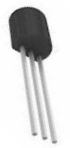﻿ N-Channel JFET Basics# N-channel JFET BasicsA N-Channel JFET is a JFET whose channel is composed of primarily electrons as the charge carrier. This means that when the transistor is turned on, it is primarily the movement of electrons which constitutes the current flow.

This is in contrast to P-Channel JFETs, whose channel is composed primarily of holes, which constitute the current flow.

A N-Channel JFET is composed of a gate, a source and a drain terminal.It is made with an N-type silicon channel that contains 2 P-type silicon terminals placed on either side. The gate lead is connected to the p-type terminals, while the drain and source leads are connected to either ends of the N-type channel.

When no voltage is applied to the gate of a N-Channel JFET, current flows freely through the central N-channel. This is why JFETs are referred to as "normally on" devices. Without any applied to the gate terminal of the transistor, they conduct current across from drain-source region.

### How a N-Channel JFET Works

This is a typical diagram you would see of voltage biasing of a N-channel JFET. This diagram also serves to show you all the parts of a N-channel JFET.### How to Turn on an N-Channel JFET

To turn on an N-channel JFET, apply a positive voltage +VDD to the drain terminal of the transistor with no voltage applied to the gate terminal of the transistor. This will allow a current to flow through the drain-source channel. If the gate voltage, VG, is 0V, the drain current is at its largest value for safe operation, and the JFET is in the ON active region.

So with a sufficient positive voltage, VDD, and no voltage (0V) applied to the base, the N-channel JFET is in maximum operation and has the largest current.

### How to Turn Off an N-Channel JFET

To turn off the N-channel JFET, there are 2 steps you can take. You can either cut off the bias positive voltage, VDD, that powers the drain. Or you can apply a negative voltage to the gate. When a negative voltage is applied to the gate, the drain current is reduced. As the gate voltage, VG, becomes more negative, the current lessens until cutoff, which is when then JFET is in the OFF region.

### Characteristics Curve of a N-Channel JFET

The characteristics curve of an N channel JFET transistor shown below is the the graph of the drain current, ID versus the gate-source voltage, VGS.

This curve represents the transconductance, or simply the gain, of the transistor.

The transconductance of a transistor really means the gain of the transistor.

So this transconductance characteristics below shows the gain of the transistor, how much current the transistor outputs based on the voltage input into the gate terminal. Remember that gain is the the output over the input. The input is how much voltage is fed to the gate terminal. The output is how much current the transistor outputs.You can see based on this N channel JFET transconductance curve that as the negative voltage to the gate increases, the gain decreases. You can see that the gain, the current ID output by the transistor, is highest when the voltage fed to the gate terminal is 0V. As we increase this voltage (negatively), again, as stated, the gain decreases.

This transconductance curve is important because it shows the operation of a N channel JFET.

You can also see that the transconductance curve, as for all semiconductor devices, is nonlinear, for most of the curve, meaning changes to VGS do not directly (linearly) increase or decrease drain current, ID, even though this is a lesser issue.

The big point is that, an N-Channel JFET turns on by having a positive voltage applied to the drain terminal of the transistor and ideally no voltage applied to the gate terminal. The transistor circuit shuts off by taking in a negative gate voltage, VGS, greater than about -4V or so. The transistor is in its fully conductive state and is in maximum operation when the voltage at the gate terminal is 0V. As we increase the amount of negative voltage the gate terminal receives, the transistor becomes less conductive. Once the negative voltage reaches a certain threshold, the N channel JFET circuit stops conducting altogether across the drain-source terminal.

The Regions that make up a transconductance curve are the following:

Cutoff Region- This is the region where the JFET transistor is off, meaning no drain current, I D flows from drain to source.

Ohmic Region- This is the region where the JFET transistor begins to show some resistance to the drain current, Id that is beginning to flow from drain to source. This is the only region in the curve where the response is linear.

Saturation Region- This is the region where the JFET transistor is fully operation and maximum current, for the voltage, VGS, that is supplied is flowing. During this region, the JFET is On and active.

Breakdown Region- This is the region where the voltage, VDD that is supplied to the drain of the transistor exceeds the necessary maximum. At this point, the JFET loses its ability to resist current because too much voltage is applied across its drain-source terminals. The transistor breaks down and current flows from drain to source.

Related Resources